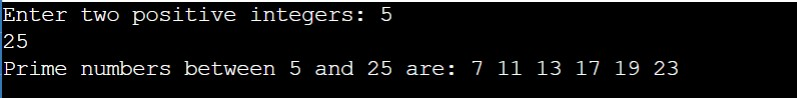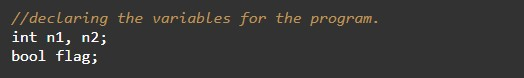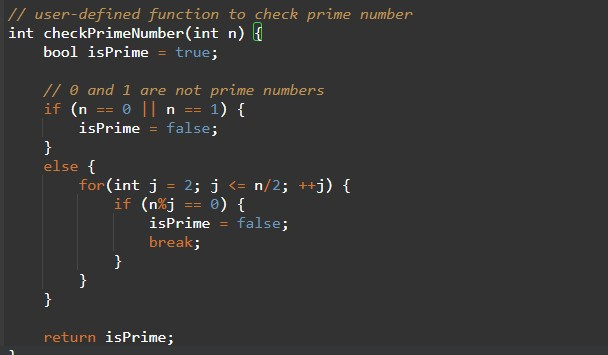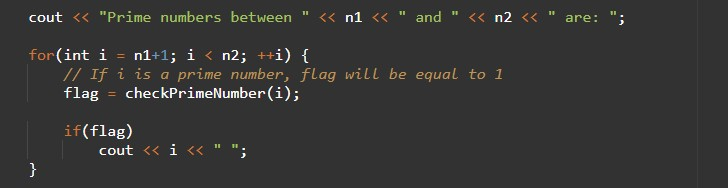# C++ Program to Display Prime Numbers Between Two Numbers Using Functions

In this tutorial you will learn about the C++ Program to Display Prime Numbers Between Two Numbers Using Functions and its application with practical example.

## C++ Program to Display Prime Numbers Between Two Numbers Using Functions

In this tutorial, we will learn to create a C++ program that will Display Prime Numbers Between Two Numbers Using Functions using C++ programming.

## Prerequisites

Before starting with this tutorial we assume that you are best aware of the following C++ programming topics:

• Operators in C++ Programming.
• Basic Input and Output function in C++ Programming.
• Basic C++ programming.
• For loop in C++ programming.
• Conditional Statements in C++ programming.
• Arithmetic operations in C++ Programming.

## Display Prime Numbers Between Two Numbers Using Functions:-

In today’s program, we will take the input range from the user to print the prime numbers between them. The prime numbers are those numbers that are only divisible by themselves. In c++ programming, we will check if that range has Prime Number or not and print those prime numbers.

With the help of this program, we can Display Prime Numbers Between Two Numbers Using Functions.

## Output:-In the above program, we have first initialized the required variable.• n1= it will hold the integer value of the input.
• n2 = it will hold the integer value of the input.
• flag = it will hold the boolean value.

Input message for the user for the integer value range.Program Logic Code user-defined function.Printing output prime number or not.In this tutorial we have learn about the C++ Program to Display Prime Numbers Between Two Numbers Using Functions and its application with practical example. I hope you will like this tutorial.